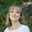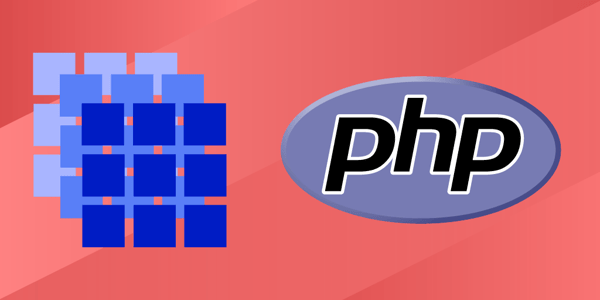# PHP arrays tutorial: Understand PHP arrays in 5 minutes

Mar 25, 2021 - 7 min readAmanda FawcettIn computer science, an array is a data structure that stores multiple values in a single variable. Think of this like a list of values. Arrays are a powerful tool for storing variables that you can access easily, as you access values by referring to their index number.

In this tutorial, we will introduce you to PHP arrays. We’ll show you how to write them and work with them. We’ll wrap up with a few hands-on problems.

Today’s tutorial at a glance:

Simply put, an array in PHP is a list, much like how in In JavaScript, an array is a list of values. For example, you could create an array of integers, like [1, 2, 3, 4, 5] or of a mixed data types, like [false, 40, 'purple', $object, 'puppy']. This makes it possible to hold multiple values under a shared name that can be accessed by referring to an index number, with the first element at index 0.As you can see, the total number of elements in an array is the length of an array. With arrays, length is dynamic and can be changed over time. PHP treats arrays a bit differently than you might be used to. In PHP, there are multiple kinds of arrays that keep different data types. PHP offers more structured arrays where we can keep sets of “key-value” pairs. Here are three types of arrays we can use in PHP: • Indexed/numeric arrays: arrays with a numeric index • Associative arrays: arrays with named keys • Multidimensional arrays: arrays that hold one or more arrays Associative arrays in PHP give us more context and information, making it very useful for web development and other programming needs. All PHP arrays are associative in nature, and you may already be used to this style. In Python, for example, these are called dictionaries, and in JavaScript, we could code the same behavior with objects. ## How to declare arrays in PHP Before the release of PHP 5.4, we create a new array using the array() function. <?php$foo = array(1, 2, 3); // An array of integers created using array fucntion
$bar = ["A", true, 123 => 5]; // Short array syntax, PHP 5.4+ echo$bar; // Returns "A"
echo "\n";
echo $bar; // Returns 1 for true echo "\n"; echo$bar; // Returns 5
// echo $bar; // uncommenting this line will give an error because 1234 is inaccessable ?> Note: We can also declare them with square brackets [ ]. The comma after the last element is optional, and it is usually done for single-line arrays like array(1, 2). The PHP list() function can also be used to assign variables in a shorter way. // define array$array = ['a', 'b', 'c'];

// without list()
$a =$array;
$b =$array;
$c =$array;

// with list()
list($a,$b, $c) =$array;


Tip: list() also works well with foreach to make the construction easier.

$arrays = [[1, 2], [3, 4], [5, 6]]; foreach ($arrays as list($a,$b)) {
$c =$a + $b; echo($c . ', '); // 3, 7, >11,
}


### Indexed/Numeric Arrays

Numeric or indexed arrays can store numbers, strings, or objects as array elements, and their index is represented by a number. Take a look at this code example to understand its syntax and run it to see the output.

<html>
<body>

<?php
/* First way to create array. */
$numbers = array( 1, 2, 3, 4, 5); foreach($numbers as $value ) { echo "Value is$value <br />";
}

/* Second way to create array. */
$numbers = "one";$numbers = "two";
$numbers = "three";$numbers = "four";
$numbers = "five"; foreach($numbers as $value ) { echo "Value is$value <br />";
}
?>

</body>
</html>

### Associative Arrays

As we discussed before, associated arrays are a lot like indexed arrays, but they are different in terms of their index. Associative arrays in PHP have their index as a string in order to create an association between key-value pairs.

For example, let’s use employees’ names as the keys in an associative array with salaries as the value.

<html>
<body>

<?php
/* First way to create array. */
$salaries = array("mark" => 2000, "janet" => 1000, "asma" => 500); echo "Salary of mark is ".$salaries['mark'] . "<br />";
echo "Salary of janet is ".  $salaries['janet']. "<br />"; echo "Salary of asma is ".$salaries['asma']. "<br />";

/* Second way to create array. */
$salaries['mark'] = "high";$salaries['janet'] = "medium";
$salaries['asma'] = "low"; echo "Salary of mark is ".$salaries['mark'] . "<br />";
echo "Salary of janet is ".  $salaries['janet']. "<br />"; echo "Salary of asma is ".$salaries['asma']. "<br />";
?>

</body>
</html>

### Multidimensional Arrays

In a multidimensional array, each element in the main array is also an array. Values in a multidimensional array are accessed using multiple indexes. The syntax declaration of 2-D arrays is as follows:

 $arrayName = array(array(), array()......array())  Let’s look at an example of a complex multidimensional array. It is an indexed array containing associative arrays. <?php // Define a multidimensional array$economy = array(
array(
"country" => "Germany",
"currency" => "Euro",
),
array(
"country" => "Switzerland",
"currency" => "Swiss Franc",
),
array(
"country" => "England",
"currency" => "Pound",
)
);

echo "Currency of Germany is: " . $economy["currency"]; // Access array at  index ?> ### Keep the learning going. Learn PHP without scrubbing through videos or documentation. Educative’s text-based courses are easy to skim and feature live coding environments, making learning quick and efficient. Learn PHP from Scratch ## PHP array functions There are a lot of different things we can do to arrays in PHP. We use array functions to manipulate, modify, and use our arrays in a meaningful way. Let’s look at some common operations. Below, we’ve listed 10 common array functions, but there are dozens more than you can use. • array_keys: return all the keys of an array • in_array: checks if a value exists in an array • array_reverse: Return an array with elements in reverse order • array_unshift: prepend one or more elements to the front of an array • array_pop: remove the element off the end of array • array_push: remove one or more elements onto the end of array • array_diff_assoc: computes the difference of arrays with additional index check • array_map: applies the callback to the elements of the given arrays • array_merge: merge one or more arrays • array_filter: filters array elements with a callback function ### Output a structured view of arrays To print an array in a readable format we use the following: print_r($arrayName)


This will print our keys and their associated values.

<?php
$fruits = array("Type"=>"Citrus",1=>"Orange",2=>"Grapefruit",3=>"Lemon");//initializing associative array print_r($fruits);
?>

### Get the length of an array

To print the length of our arrays, we can use the count() function.

count ( Countable|array $value , int$mode = COUNT_NORMAL ) : int

<?php
$food = array('fruits' => array('orange', 'banana', 'apple'), 'veggie' => array('carrot', 'collard', 'pea')); // recursive count echo count($food, COUNT_RECURSIVE); // output 8

// normal count
echo count($food); // output 2 ?> Recursive Count Function ### Accessing array elements Array elements can be accessed using array[key] . <?php$array = array(
"foo" => "bar",
42    => 24,
"multi" => array(
"dimensional" => array(
"array" => "foo"
)
)
);

var_dump($array["foo"]); var_dump($array);
var_dump($array["multi"]["dimensional"]["array"]); ?> ### Return all the values of an array We can return the values of our array with the PHP function array_values. array_values ( array$array ) : array

<?php
$array = array("size" => "M", "color" => "yellow"); print_r(array_values($array));
?>

### Looping through arrays

When working with arrays, it’s common to iterate through an array and perform something on the elements, such as add them or display them. The most common way to iterate through an array in PHP is using foreach.

You can also use a for loop, but foreach is generally easier to write and read.

foreach ($array as$key => $value) { echo "Key is$key.";
echo "Value is $value."; } Basic syntax of foreach with array ## Hands-on PHP array challenges Let’s put what we’ve learned into practice with a few hands-on challenges. Our first challenge is to find the maximum value of our array. You should write a method named findMaxVal() to find the maximum integer value stored in an array of any size. Your input is an array passed as a parameter, and your output should be the maximum value found in the array as an integer. Try it yourself below before checking the solution. Sample Input: {2, 9, 6, 17, 15} Sample Output: 17 <?php //Returns maximum value from Array passed as parameter function findMaxVal($arr)
{

return -1; //change this line to return the maximum value

}
?>

Our second challenge is to print a two-dimensional array of size n x n as shown below in the form of a matrix.

Your input will be an integer, for example $n = 3;, and your output should print a matrix on the console. Print an array of size 3 x 3 as: • The diagonal of the array should be filled with 0. • The lower side should be filled with -1s. • The upper side should be filled with 1s. Make sure to put spaces between the values and print each row in the next line. In order to move a value to the next line you can use PHP_EOL. 0 1 1 \n-1 0 1 \n-1 -1 0 \n  Sample Output Matrix Try it yourself below before checking the solution. <?php function printMat($n) {
//write the code for making and printing the matrix here
//use can use \n to move numers to next line in the matrix
//use " " to add space between numbers in matrix
echo "not complete";//comment out this line when you start writing code
}
?>

## What to learn next

You should now have a good idea how arrays work in PHP, and you’re ready to tackle more advanced concepts. Next we recommend learning the following:

• Combining array functions
• Converting to arrays
• Indexed arrays without key
• Defining classes in PHP

To get started with these concepts and more, check out Educative’s course Learn PHP from Scratch. This highly interactive course introduces you to fundamental concepts of PHP. It begins with a simple Hello world program and proceeds on to cover common concepts such as Conditional Statements, and Loop Statements.

By the time you’re done, you’ll have a good grip on the basics of PHP, and will be ready to study advanced concepts.

Happy learning!